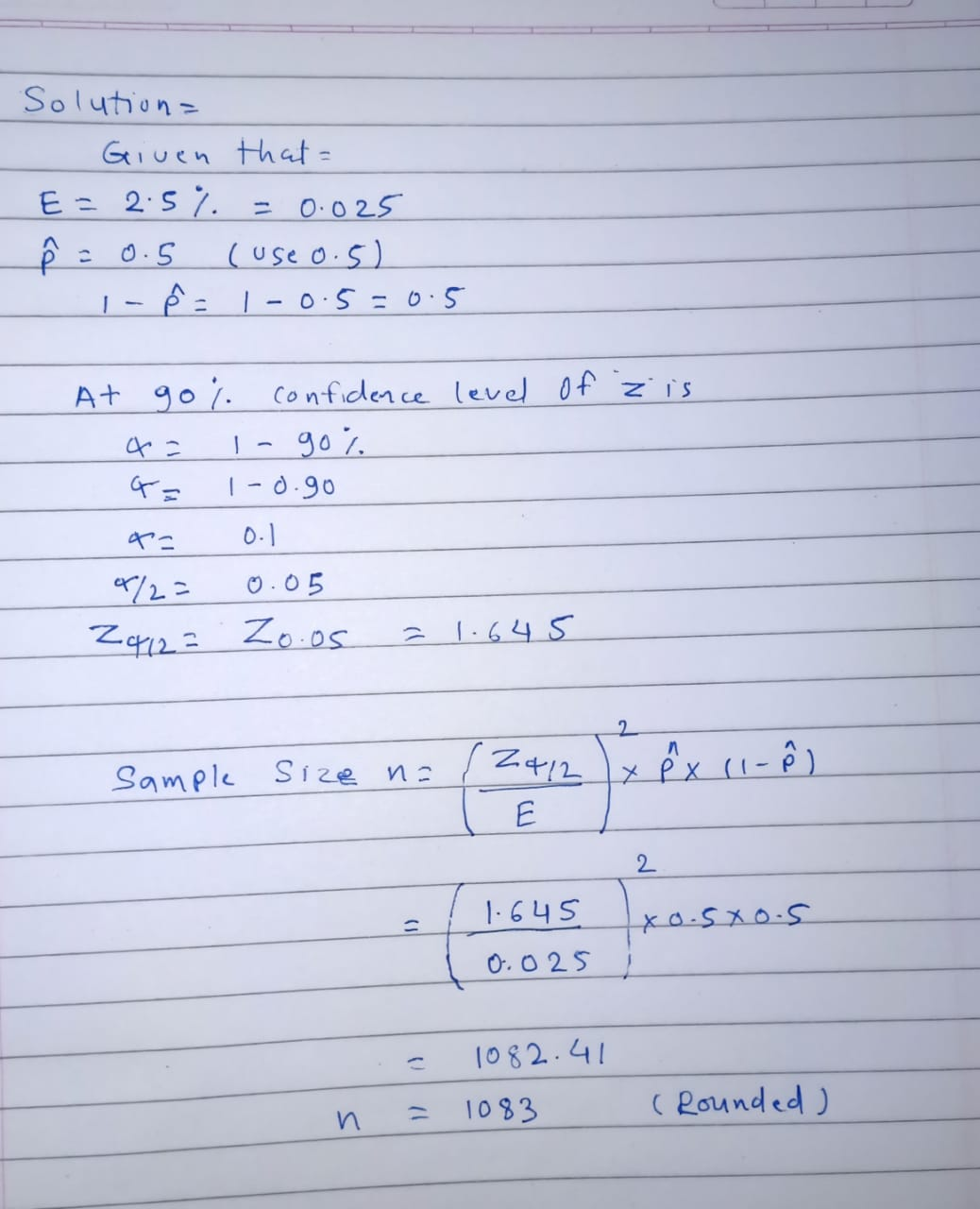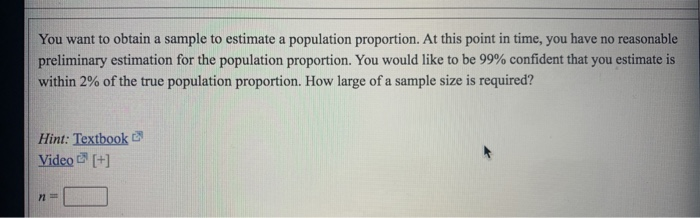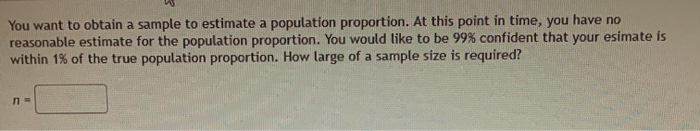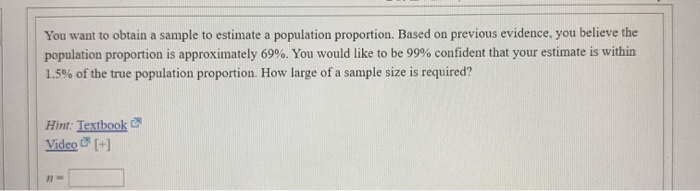# You want to obtain a sample to estimate a population proportion. At this point in time,...

You want to obtain a sample to estimate a population proportion. At this point in time, you have no reasonable preliminary estimation for the population proportion. You would like to be 90% confident that you estimate is within 2.5% of the true population proportion. How large of a sample size is required?#### Earn Coin

Coins can be redeemed for fabulous gifts.

Similar Homework Help Questions
• ### You want to obtain a sample to estimate a population proportion. At this point in time,...

You want to obtain a sample to estimate a population proportion. At this point in time, you have no reasonable preliminary estimation for the population proportion. You would like to be 98% confident that you estimate is within 2% of the true population proportion. How large of a sample size is required?

• ### You want to obtain a sample to estimate a population proportion. At this point in time,...

You want to obtain a sample to estimate a population proportion. At this point in time, you have no reasonable preliminary estimation for the population proportion. You would like to be 99% confident that you estimate is within 3% of the true population proportion. How large of a sample size is required? n =

• ### You want to obtain a sample to estimate a population proportion. At this point in time,...You want to obtain a sample to estimate a population proportion. At this point in time, you have no reasonable preliminary estimation for the population proportion. You would like to be 99% confident that you estimate is within 2% of the true population proportion. How large of a sample size is required? Hint: Textbook Video [+] N-

• ### You want to obtain a sample to estimate a population proportion. At this point in time,...You want to obtain a sample to estimate a population proportion. At this point in time, you have no reasonable estimate for the population proportion. You would like to be 99% confident that your esimate is within 1% of the true population proportion. How large of a sample size is required? n =

• ### You want to obtain a sample to estimate a population proportion. At this point in time,...

You want to obtain a sample to estimate a population proportion. At this point in time, you have no reasonable estimate for the population proportion. You would like to be 95% confident that you esimate is within 4% of the true population proportion. How large of a sample size is required? n=? Do not round mid-calculation. However, use a critical value accurate to three decimal places.

• ### You want to obtain a sample to estimate a population proportion. Based on previous evidence, you...

You want to obtain a sample to estimate a population proportion. Based on previous evidence, you believe the population proportion is approximately p = 0.15. You would like to be 98% confident that your esimate is within 2.5% of the true population proportion. How large of a sample size is required? Hint: Video [+] n =

• ### You want to obtain a sample to estimate a population proportion. Based on previous evidence, you...

You want to obtain a sample to estimate a population proportion. Based on previous evidence, you believe the population proportion is approximately 35%. You would like to be 99% confident that your estimate is within 1% of the true population proportion. How large of a sample size is required? n=

• ### You want to obtain a sample to estimate a population proportion. Based on previous evidence, you...

You want to obtain a sample to estimate a population proportion. Based on previous evidence, you believe the population proportion is approximately 17%. You would like to be 99% confident that your estimate is within 1% of the true population proportion. How large of a sample size is required? n =

• ### You want to obtain a sample to estimate a population proportion. Based on previous evidence, you...

You want to obtain a sample to estimate a population proportion. Based on previous evidence, you believe the population proportion is approximately p∗=16%p∗=16%. You would like to be 99% confident that your estimate is within 5% of the true population proportion. How large of a sample size is required?

• ### You want to obtain a sample to estimate a population proportion. Based on previous evidence, you...You want to obtain a sample to estimate a population proportion. Based on previous evidence, you believe the population proportion is approximately 69%. You would like to be 99% confident that your estimate is within 1.5% of the true population proportion. How large of a sample size is required? Hint: Textbook Video [+]